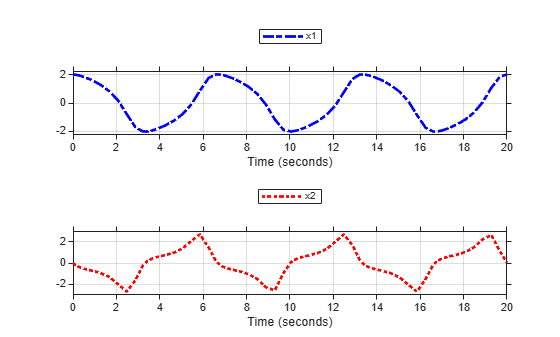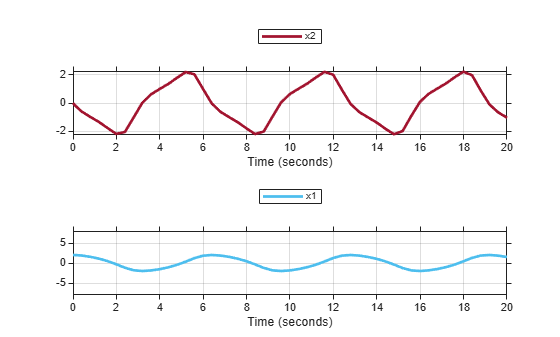# plotOnSubPlot

Plot signals on `Simulink.sdi.CustomSnapshot` object subplots

## Syntax

```snap.plotOnSubPlot(row,column,signal,plot) ```

## Description

`snap.plotOnSubPlot(row,column,signal,plot)` plots the `signal` on the subplot in the `Simulink.sdi.CustomSnapshot` object, `snap`, specified by `row` and `column` when `plot` is `true`. When `plot` is `false`, `plotOnSubPlot` clears the `signal` from the subplot.

## Input Arguments

expand all

Row for subplot on which you want to plot a signal. Specify `row` as a value from 1 through 8, inclusive.

Example: `2`

Column for subplot on which you want to plot a signal. Specify `column` as a value from 1 through 8, inclusive.

Example: `3`

Signal ID or `Simulink.sdi.Signal` object corresponding to the signal you want to plot.

Example: `sigID`

Logical indicator of whether to plot or clear the signal from the subplot.

• `true` – Plot the signal.

• `false` – Clear the signal.

Example: `true`

Data Types: `logical`

## Examples

expand all

This example shows how to copy view settings from one run to another and how to create figures using the `Simulink.sdi.CustomSnapshot` object.

Simulate Your Model and Get a Run Object

Configure the `vdp` model to save output data, and run a simulation to create data.

```load_system('vdp') set_param('vdp','SaveFormat','Dataset','SaveOutput','on') set_param('vdp/Mu','Gain','1'); sim('vdp');```

Use the Simulation Data Inspector programmatic interface to access the run data.

```runIndex = Simulink.sdi.getRunCount; runID = Simulink.sdi.getRunIDByIndex(runIndex); vdpRun = Simulink.sdi.getRun(runID);```

Modify Signal View Settings

Use the `Simulink.sdi.Run` object to access signals in the run. Then, modify the signal view settings. This example specifies the line color and style for each signal. The view settings for the run comprise the view settings for each signal and view settings specified for the plot area.

```sig1 = vdpRun.getSignalByIndex(1); sig2 = vdpRun.getSignalByIndex(2); sig1.LineColor = [0 0 1]; sig1.LineDashed = '-.'; sig2.LineColor = [1 0 0]; sig2.LineDashed = ':';```

Capture a Snapshot from the Simulation Data Inspector

Create a `Simulink.sdi.CustomSnapshot` object and use the `Simulink.sdi.snapshot` function to programmatically capture a snapshot of the contents of the Simulation Data Inspector.

`snap = Simulink.sdi.CustomSnapshot;`

You can use properties of the `Simulink.sdi.CustomSnapshot` object to configure the plot settings, like the subplot layout and axis limits, and to plot signals. When you use a `Simulink.sdi.CustomSnapshot` object to create your figure, these plot settings do not affect the Simulation Data Inspector.

```snap.Rows = 2; snap.YRange = {[-2.25 2.25],[-3 3]}; snap.plotOnSubPlot(1,1,sig1,true) snap.plotOnSubPlot(2,1,sig2,true)```

Use `Simulink.sdi.snapshot` to generate the figure you specified in the properties of the `Simulink.sdi.CustomSnapshot` object.

`fig = Simulink.sdi.snapshot("from","custom","to","figure","settings",snap);`Copy the View Settings to a New Simulation Run

Simulate the model again, with a different `Mu` value. Use the Simulation Data Inspector programmatic interface to access the simulation data.

```set_param('vdp/Mu','Gain','5') sim('vdp'); runIndex2 = Simulink.sdi.getRunCount; runID2 = Simulink.sdi.getRunIDByIndex(runIndex2); run2 = Simulink.sdi.getRun(runID2);```

To create a plot of the new output data that looks like the one you created in the previous step, you can copy the view settings to the run in a single line of code using `Simulink.sdi.copyRunViewSettings`. The `Simulink.sdi.copyRunViewSettings` function does not automatically update plot settings in `Simulink.sdi.CustomSnapshot` objects, so specify the `plot` input as `false`.

`sigIDs = Simulink.sdi.copyRunViewSettings(runID,runID2,false);`

Capture a Snapshot of the New Simulation Run

Use the `Simulink.sdi.CustomSnapshot` object to capture a snapshot of the new simulation run. First, clear the signals from the subplots. Then, plot the signals from the new run and capture another snapshot.

```snap.clearSignals snap.YRange = {[-2.25 2.25],[-8 8]}; snap.plotOnSubPlot(1,1,sigIDs(1),true) snap.plotOnSubPlot(2,1,sigIDs(2),true) fig = snap.snapshot("to","figure");```